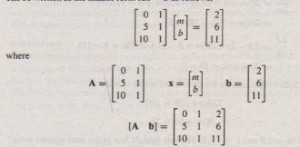The Least Squares Method
Suppose we have the following three data points, and we want to find the straight line Y = mx +b that best fits the data in some sense.(a) Find the coefficients m and b by using the least squares criterion. (b) Find the
coefficients by using MATLAB to solve the three equations (one for each data point) for
the two unknowns m and b. Compare the answers from (a) and (b).
• Solution
(a) Because two points define a straight line, unless we are extremely lucky, our data points will not lie on the same straight line. A common criterion for obtaining the straight line that best fits the data is the least squares criterion. According to this criterion, the line that minimizes J, the sum of the squares of the vertical differences between the line and the data points, is the “best” fit (see Figure 6.5-1). Here J isSubstituting the data values (Xi, Yi), this expression becomes

J = (Om + b – 2)2 + (5m + b – 6)2 + (10m + b – 11)2

You can use the frninsearch command to find the values of m and b that minimize J. On the other hand” if you are familiar with calculus, you know that the values of m and b that minimize J are found by setting the partial derivatives aJ jam and aJ lab

equal to zero:These give the following equations for the two unknowns m and b:

250m + 30b = 280
30m +6b = 38

The solution is m = 0.9 and b = 11/6. The best straight line in the least squares sense is y = 0.9x + 11/6 = 0.9x + 1.8333. It appears in Figure 6.5-2, along with the data points.
(b) Evaluating the equation y = mx + b at each data point gives the following three
equations:

Om+b=2
5m +b = 6
10m +b = II

This set is over-determined because it has more equations than unknowns. These equations can be written in the matrix form Ax = b as followsBecause we can find a nonzero 2 x 2 determinant in A, its rank is two. However IA b] = -5 ¥- 0, so its rank is three. Thus no exact solution exists for 111 and b. The following MATLAB session uses left division.
»A = (0,1; 5, 1; 10, 1] ;
»b = [2;6;11];
»rank(A)
ans
2

»rank ([A b])
ans =
3
»A\b
ans
0.9000
1. 8333
This result agrees with the least squares solution obtained previously: 1/1 = 0.9, b =
11/6 = 1.8333.
If we now type A * ans, MATLAB yields this result:
ans
1. 833
6.333
10.8333
These values are the y values generated by the line y = 0.9x + 1.8333 at the x data values x = 0,5, 10.These values are different from the right sides of the original three equations (6.5-;1) through (6.5-3). This result is not unexpected because the least squares solution is not an exact solution of the equations.